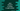# Java program to check if a number is a ugly number or not## Java program to check if a number is a ugly number or not:

In this post, we will learn how to check if a number is a ugly number or not using Java. The program will ask the user to enter a number, it will read that number and check if it is ugly or not.

Before we move to the program, let’s understand what are ugly numbers i.e. the definition of ugly numbers.

### Definition of ugly number:

A number is called a ugly number if its prime factors are 2, 3 and 5. For example, 15 is ugly number because its factors are 1 * 3 * 5. 1 is also considered as ugly number.

Let’s write a Java program that checks if a given number is ugly number or not.

### Java program to check if a number is ugly or not:

The below program takes one number as input from the user and checks if that number is ugly or not:

``````import java.util.Scanner;

public class Main {

public static void main(String[] args) {
boolean isUgly = true;
Scanner sc = new Scanner(System.in);

System.out.println("Enter a number: ");
int no = sc.nextInt();

while (no != 1) {
if (no % 5 == 0) {
no = no / 5;
} else if (no % 3 == 0) {
no = no / 3;
} else if (no % 2 == 0) {
no = no / 2;
} else {
isUgly = false;
break;
}
}

if (isUgly) {
System.out.println("It is an ugly number");
} else {
System.out.println("It is not an ugly number");
}

}
}``````

Here,

• isUgly is a boolean. It is initialized as true. It is assigned false if the given number is not ugly.
• The Scanner variable sc is used to read the user input number
• The while loop runs until the value of the number is 1. Inside this loop, it divides the number by 5, 3 or 2. If it can’t divide it by any of these numbers, it assigns false to isUgly and exits from the loop.
• The last if-else block is checking the value of isUgly and based on the value, it prints a message.

If you run this program, it will print output as like below:

``````Enter a number:
100
It is an ugly number``````

Let’s write a Java program to print all ugly numbers from 1 to 100. We can move the above code that checks if a number is ugly or not to a different method and use that method for all numbers from 1 to 100 using a loop.

``````public class Main {

private static boolean isUgly(int no) {
while (no != 1) {
if (no % 5 == 0) {
no = no / 5;
} else if (no % 3 == 0) {
no = no / 3;
} else if (no % 2 == 0) {
no = no / 2;
} else {
return false;
}
}
return true;
}

public static void main(String[] args) {
for (int i = 1; i <= 100; i++) {
if (isUgly(i)) {
System.out.println(i);
}
}
}
}``````

Here,

• isUgly method checks if a number is ugly or not. It takes one integer value and returns one boolean. true if the integer is ugly, false otherwise.
• It uses a while loop in a similar way as we did for the previous program.
• Inside main, we are using a for loop that runs from 1 to 100 that checks and prints all ugly numbers.

If you run this program, it will print all ugly numbers from 1 to 100:

``````1
2
3
4
5
6
8
9
10
12
15
16
18
20
24
25
27
30
32
36
40
45
48
50
54
60
64
72
75
80
81
90
96
100``````

Or, you can use System.out.print(i + ” ”); to print these in one line:

``1 2 3 4 5 6 8 9 10 12 15 16 18 20 24 25 27 30 32 36 40 45 48 50 54 60 64 72 75 80 81 90 96 100 ``